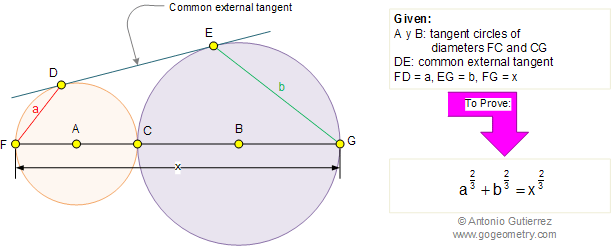Problem 270. Tangent Circles, Common external tangent, Fractional exponents

 In the figure below, A and B are tangent circles of diameters FC and CG respectively. If DE is the common external tangent, FD = a, EG = b, and FG = x, prove that: a2/3 + b2/3 = x2/3. View or post a solution.Home | Sitemap | Geometry | Problems | 261-270 | Search | Email | By Antonio Gutierrez